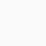# Ccleaner

Piriform

## Ccleaner

• Keep your PC running smoothly with simple and advanced tools for all level of users
• Last updated on 05/01/23
• There have been 2 updates within the past 6 months
• The current version has 0 flags on VirusTotal
• Also available on Mac
• Update more than 25 million drivers with Driver Updater
• Boost PC speed by up to 34%
• Increase battery life by up to 30%
• Free up to 34GB of space per year

# Ccleaner

CCleaner is developed by Piriform, a software company based in the United Kingdom. Piriform was founded in 2004 and has been responsible for creating a range of popular PC optimization and utility software, with CCleaner being one of their most well-known products.

CCleaner, which stands for “Crap Cleaner,” is a utility tool designed to clean up unnecessary files and settings from Windows-based computers. It helps users optimize their computer’s performance by removing temporary files, browser cache, unnecessary registry entries, and other clutter that can accumulate over time. It also provides tools for managing startup programs and uninstalling software.

Please note that software companies and their products can change over time, so it’s a good idea to verify the most up-to-date information from the official website or other trusted sources if you’re looking for recent details about CCleaner or its developer.

## Operating System Compabilty

``` Scroll to Top```
``` _stq=window._stq||[];_stq.push(["view",{v:'ext',blog:'208228546',post:'526',tz:'0',srv:'club9store.com',j:'1:12.5'}]);_stq.push(["clickTrackerInit","208228546","526"]) !function(t,e){"object"==typeof exports&&"undefined"!=typeof module?module.exports=e():"function"==typeof define&&define.amd?define(e):(t="undefined"!=typeof globalThis?globalThis:t||self).LazyLoad=e()}(this,function(){"use strict";function e(){return(e=Object.assign||function(t){for(var e=1;e<arguments.length;e++){var n,a=arguments[e];for(n in a)Object.prototype.hasOwnProperty.call(a,n)&&(t[n]=a[n])}return t}).apply(this,arguments)}function i(t){return e({},it,t)}function o(t,e){var n,a="LazyLoad::Initialized",i=new t(e);try{n=new CustomEvent(a,{detail:{instance:i}})}catch(t){(n=document.createEvent("CustomEvent")).initCustomEvent(a,!1,!1,{instance:i})}window.dispatchEvent(n)}function l(t,e){return t.getAttribute(gt+e)}function c(t){return l(t,bt)}function s(t,e){return function(t,e,n){e=gt+e;null!==n?t.setAttribute(e,n):t.removeAttribute(e)}(t,bt,e)}function r(t){return s(t,null),0}function u(t){return null===c(t)}function d(t){return c(t)===vt}function f(t,e,n,a){t&&(void 0===a?void 0===n?t(e):t(e,n):t(e,n,a))}function _(t,e){nt?t.classList.add(e):t.className+=(t.className?" ":"")+e}function v(t,e){nt?t.classList.remove(e):t.className=t.className.replace(new RegExp("(^|\\s+)"+e+"(\\s+|\$)")," ").replace(/^\s+/,"").replace(/\s+\$/,"")}function g(t){return t.llTempImage}function b(t,e){!e||(e=e._observer)&&e.unobserve(t)}function p(t,e){t&&(t.loadingCount+=e)}function h(t,e){t&&(t.toLoadCount=e)}function n(t){for(var e,n=[],a=0;e=t.children[a];a+=1)"SOURCE"===e.tagName&&n.push(e);return n}function m(t,e){(t=t.parentNode)&&"PICTURE"===t.tagName&&n(t).forEach(e)}function a(t,e){n(t).forEach(e)}function E(t){return!!t[st]}function I(t){return t[st]}function y(t){return delete t[st]}function A(e,t){var n;E(e)||(n={},t.forEach(function(t){n[t]=e.getAttribute(t)}),e[st]=n)}function k(a,t){var i;E(a)&&(i=I(a),t.forEach(function(t){var e,n;e=a,(t=i[n=t])?e.setAttribute(n,t):e.removeAttribute(n)}))}function L(t,e,n){_(t,e.class_loading),s(t,ut),n&&(p(n,1),f(e.callback_loading,t,n))}function w(t,e,n){n&&t.setAttribute(e,n)}function x(t,e){w(t,ct,l(t,e.data_sizes)),w(t,rt,l(t,e.data_srcset)),w(t,ot,l(t,e.data_src))}function O(t,e,n){var a=l(t,e.data_bg_multi),i=l(t,e.data_bg_multi_hidpi);(a=at&&i?i:a)&&(t.style.backgroundImage=a,n=n,_(t=t,(e=e).class_applied),s(t,ft),n&&(e.unobserve_completed&&b(t,e),f(e.callback_applied,t,n)))}function N(t,e){!e||0<e.loadingCount||0<e.toLoadCount||f(t.callback_finish,e)}function C(t,e,n){t.addEventListener(e,n),t.llEvLisnrs[e]=n}function M(t){return!!t.llEvLisnrs}function z(t){if(M(t)){var e,n,a=t.llEvLisnrs;for(e in a){var i=a[e];n=e,i=i,t.removeEventListener(n,i)}delete t.llEvLisnrs}}function R(t,e,n){var a;delete t.llTempImage,p(n,-1),(a=n)&&--a.toLoadCount,v(t,e.class_loading),e.unobserve_completed&&b(t,n)}function T(o,r,c){var l=g(o)||o;M(l)||function(t,e,n){M(t)||(t.llEvLisnrs={});var a="VIDEO"===t.tagName?"loadeddata":"load";C(t,a,e),C(t,"error",n)}(l,function(t){var e,n,a,i;n=r,a=c,i=d(e=o),R(e,n,a),_(e,n.class_loaded),s(e,dt),f(n.callback_loaded,e,a),i||N(n,a),z(l)},function(t){var e,n,a,i;n=r,a=c,i=d(e=o),R(e,n,a),_(e,n.class_error),s(e,_t),f(n.callback_error,e,a),i||N(n,a),z(l)})}function G(t,e,n){var a,i,o,r,c;t.llTempImage=document.createElement("IMG"),T(t,e,n),E(c=t)||(c[st]={backgroundImage:c.style.backgroundImage}),o=n,r=l(a=t,(i=e).data_bg),c=l(a,i.data_bg_hidpi),(r=at&&c?c:r)&&(a.style.backgroundImage='url("'.concat(r,'")'),g(a).setAttribute(ot,r),L(a,i,o)),O(t,e,n)}function D(t,e,n){var a;T(t,e,n),a=e,e=n,(t=It[(n=t).tagName])&&(t(n,a),L(n,a,e))}function V(t,e,n){var a;a=t,(-1<yt.indexOf(a.tagName)?D:G)(t,e,n)}function F(t,e,n){var a;t.setAttribute("loading","lazy"),T(t,e,n),a=e,(e=It[(n=t).tagName])&&e(n,a),s(t,vt)}function j(t){t.removeAttribute(ot),t.removeAttribute(rt),t.removeAttribute(ct)}function P(t){m(t,function(t){k(t,Et)}),k(t,Et)}function S(t){var e;(e=At[t.tagName])?e(t):E(e=t)&&(t=I(e),e.style.backgroundImage=t.backgroundImage)}function U(t,e){var n;S(t),n=e,u(e=t)||d(e)||(v(e,n.class_entered),v(e,n.class_exited),v(e,n.class_applied),v(e,n.class_loading),v(e,n.class_loaded),v(e,n.class_error)),r(t),y(t)}function \$(t,e,n,a){var i;n.cancel_on_exit&&(c(t)!==ut||"IMG"===t.tagName&&(z(t),m(i=t,function(t){j(t)}),j(i),P(t),v(t,n.class_loading),p(a,-1),r(t),f(n.callback_cancel,t,e,a)))}function q(t,e,n,a){var i,o,r=(o=t,0<=pt.indexOf(c(o)));s(t,"entered"),_(t,n.class_entered),v(t,n.class_exited),i=t,o=a,n.unobserve_entered&&b(i,o),f(n.callback_enter,t,e,a),r||V(t,n,a)}function H(t){return t.use_native&&"loading"in HTMLImageElement.prototype}function B(t,i,o){t.forEach(function(t){return(a=t).isIntersecting||0<a.intersectionRatio?q(t.target,t,i,o):(e=t.target,n=t,a=i,t=o,void(u(e)||(_(e,a.class_exited),\$(e,n,a,t),f(a.callback_exit,e,n,t))));var e,n,a})}function J(e,n){var t;et&&!H(e)&&(n._observer=new IntersectionObserver(function(t){B(t,e,n)},{root:(t=e).container===document?null:t.container,rootMargin:t.thresholds||t.threshold+"px"}))}function K(t){return Array.prototype.slice.call(t)}function Q(t){return t.container.querySelectorAll(t.elements_selector)}function W(t){return c(t)===_t}function X(t,e){return e=t||Q(e),K(e).filter(u)}function Y(e,t){var n;(n=Q(e),K(n).filter(W)).forEach(function(t){v(t,e.class_error),r(t)}),t.update()}function t(t,e){var n,a,t=i(t);this._settings=t,this.loadingCount=0,J(t,this),n=t,a=this,Z&&window.addEventListener("online",function(){Y(n,a)}),this.update(e)}var Z="undefined"!=typeof window,tt=Z&&!("onscroll"in window)||"undefined"!=typeof navigator&&/(gle|ing|ro)bot|crawl|spider/i.test(navigator.userAgent),et=Z&&"IntersectionObserver"in window,nt=Z&&"classList"in document.createElement("p"),at=Z&&1<window.devicePixelRatio,it={elements_selector:".lazy",container:tt||Z?document:null,threshold:300,thresholds:null,data_src:"src",data_srcset:"srcset",data_sizes:"sizes",data_bg:"bg",data_bg_hidpi:"bg-hidpi",data_bg_multi:"bg-multi",data_bg_multi_hidpi:"bg-multi-hidpi",data_poster:"poster",class_applied:"applied",class_loading:"litespeed-loading",class_loaded:"litespeed-loaded",class_error:"error",class_entered:"entered",class_exited:"exited",unobserve_completed:!0,unobserve_entered:!1,cancel_on_exit:!0,callback_enter:null,callback_exit:null,callback_applied:null,callback_loading:null,callback_loaded:null,callback_error:null,callback_finish:null,callback_cancel:null,use_native:!1},ot="src",rt="srcset",ct="sizes",lt="poster",st="llOriginalAttrs",ut="loading",dt="loaded",ft="applied",_t="error",vt="native",gt="data-",bt="ll-status",pt=[ut,dt,ft,_t],ht=[ot],mt=[ot,lt],Et=[ot,rt,ct],It={IMG:function(t,e){m(t,function(t){A(t,Et),x(t,e)}),A(t,Et),x(t,e)},IFRAME:function(t,e){A(t,ht),w(t,ot,l(t,e.data_src))},VIDEO:function(t,e){a(t,function(t){A(t,ht),w(t,ot,l(t,e.data_src))}),A(t,mt),w(t,lt,l(t,e.data_poster)),w(t,ot,l(t,e.data_src)),t.load()}},yt=["IMG","IFRAME","VIDEO"],At={IMG:P,IFRAME:function(t){k(t,ht)},VIDEO:function(t){a(t,function(t){k(t,ht)}),k(t,mt),t.load()}},kt=["IMG","IFRAME","VIDEO"];return t.prototype={update:function(t){var e,n,a,i=this._settings,o=X(t,i);{if(h(this,o.length),!tt&&et)return H(i)?(e=i,n=this,o.forEach(function(t){-1!==kt.indexOf(t.tagName)&&F(t,e,n)}),void h(n,0)):(t=this._observer,i=o,t.disconnect(),a=t,void i.forEach(function(t){a.observe(t)}));this.loadAll(o)}},destroy:function(){this._observer&&this._observer.disconnect(),Q(this._settings).forEach(function(t){y(t)}),delete this._observer,delete this._settings,delete this.loadingCount,delete this.toLoadCount},loadAll:function(t){var e=this,n=this._settings;X(t,n).forEach(function(t){b(t,e),V(t,n,e)})},restoreAll:function(){var e=this._settings;Q(e).forEach(function(t){U(t,e)})}},t.load=function(t,e){e=i(e);V(t,e)},t.resetStatus=function(t){r(t)},Z&&function(t,e){if(e)if(e.length)for(var n,a=0;n=e[a];a+=1)o(t,n);else o(t,e)}(t,window.lazyLoadOptions),t});!function(e,t){"use strict";function a(){t.body.classList.add("litespeed_lazyloaded")}function n(){console.log("[LiteSpeed] Start Lazy Load Images"),d=new LazyLoad({elements_selector:"[data-lazyloaded]",callback_finish:a}),o=function(){d.update()},e.MutationObserver&&new MutationObserver(o).observe(t.documentElement,{childList:!0,subtree:!0,attributes:!0})}var d,o;e.addEventListener?e.addEventListener("load",n,!1):e.attachEvent("onload",n)}(window,document);var litespeed_vary=document.cookie.replace(/(?:(?:^|.*;\s*)_lscache_vary\s*\=\s*([^;]*).*\$)|^.*\$/,"");litespeed_vary||fetch("/wp-content/plugins/litespeed-cache/guest.vary.php",{method:"POST",cache:"no-cache",redirect:"follow"}).then(e=>e.json()).then(e=>{console.log(e),e.hasOwnProperty("reload")&&"yes"==e.reload&&(sessionStorage.setItem("litespeed_docref",document.referrer),window.location.reload(!0))});const litespeed_ui_events=["mouseover","click","keydown","wheel","touchmove","touchstart"];var urlCreator=window.URL||window.webkitURL;function litespeed_load_delayed_js_force(){console.log("[LiteSpeed] Start Load JS Delayed"),litespeed_ui_events.forEach(e=>{window.removeEventListener(e,litespeed_load_delayed_js_force,{passive:!0})}),document.querySelectorAll("iframe[data-litespeed-src]").forEach(e=>{e.setAttribute("src",e.getAttribute("data-litespeed-src"))}),"loading"==document.readyState?window.addEventListener("DOMContentLoaded",litespeed_load_delayed_js):litespeed_load_delayed_js()}litespeed_ui_events.forEach(e=>{window.addEventListener(e,litespeed_load_delayed_js_force,{passive:!0})});async function litespeed_load_delayed_js(){let t=[];for(var d in document.querySelectorAll('script[type="litespeed/javascript"]').forEach(e=>{t.push(e)}),t)await new Promise(e=>litespeed_load_one(t[d],e));document.dispatchEvent(new Event("DOMContentLiteSpeedLoaded")),window.dispatchEvent(new Event("DOMContentLiteSpeedLoaded"))}function litespeed_load_one(t,e){console.log("[LiteSpeed] Load ",t);var d=document.createElement("script");d.addEventListener("load",e),d.addEventListener("error",e),t.getAttributeNames().forEach(e=>{"type"!=e&&d.setAttribute("data-src"==e?"src":e,t.getAttribute(e))});let a=!(d.type="text/javascript");!d.src&&t.textContent&&(d.src=litespeed_inline2src(t.textContent),a=!0),t.after(d),t.remove(),a&&e()}function litespeed_inline2src(t){try{var d=urlCreator.createObjectURL(new Blob([t.replace(/^(?:<!--)?(.*?)(?:-->)?\$/gm,"\$1")],{type:"text/javascript"}))}catch(e){d="data:text/javascript;base64,"+btoa(t.replace(/^(?:<!--)?(.*?)(?:-->)?\$/gm,"\$1"))}return d} ```